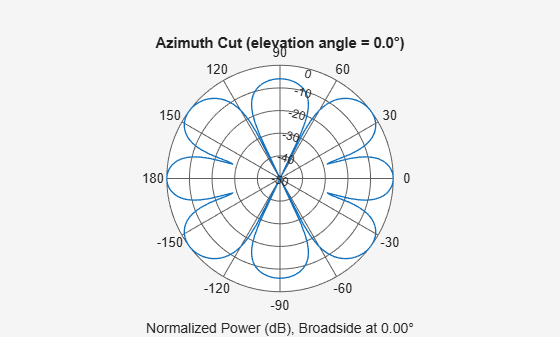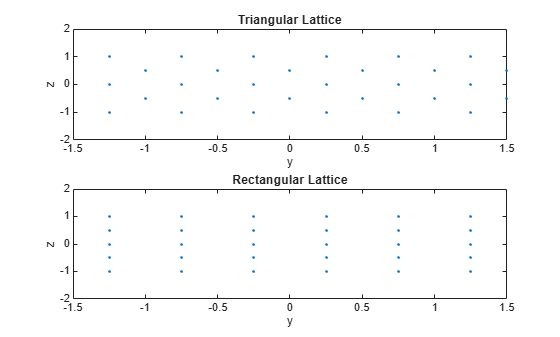# phased.URA

Uniform rectangular array

## Description

The `URA` object constructs a uniform rectangular array (URA).

To compute the response for each element in the array for specified directions:

1. Define and set up your uniform rectangular array. See Construction.

2. Call `step` to compute the response according to the properties of `phased.URA`. The behavior of `step` is specific to each object in the toolbox.

Note

Starting in R2016b, instead of using the `step` method to perform the operation defined by the System object™, you can call the object with arguments, as if it were a function. For example, ```y = step(obj,x)``` and `y = obj(x)` perform equivalent operations.

## Construction

`H = phased.URA` creates a uniform rectangular array System object, `H`. The object models a URA formed with identical sensor elements. Array elements are distributed in the yz-plane in a rectangular lattice. The array look direction (boresight) is along the positive x-axis.

`H = phased.URA(Name,Value)` creates the object, `H`, with each specified property Name set to the specified Value. You can specify additional name-value pair arguments in any order as (`Name1`,`Value1`,...,`NameN`,`ValueN`).

`H = phased.URA(SZ,D,Name,Value)` creates a URA object, `H`, with the `Size` property set to `SZ`, the `ElementSpacing` property set to `D` and other specified property Names set to the specified Values. `SZ` and `D` are value-only arguments. When specifying a value-only argument, specify all preceding value-only arguments. You can specify name-value pair arguments in any order.

## Properties

`Element`

Phased array toolbox system object

Element specified as a Phased Array System Toolbox object. This object can be an antenna or microphone element.

Default: Isotropic antenna element with default properties

`Size`

Size of array

A 1-by-2 integer vector or a single integer containing the size of the array. If `Size` is a 1-by-2 vector, the vector has the form `[NumberOfRows, NumberOfColumns]`. If `Size` is a scalar, the array has the same number of elements in each row and column. For a URA, array elements are indexed from top to bottom along a column and continuing to the next columns from left to right. In this illustration, a `'Size'` value of `[3,2]` array has three rows and two columns.Default: `[2 2]`

`ElementSpacing`

Element spacing

A 1-by-2 vector or a scalar containing the element spacing of the array, expressed in meters. If `ElementSpacing` is a 1-by-2 vector, it is in the form of `[SpacingBetweenRows,SpacingBetweenColumns]`. See Spacing Between Columns and Spacing Between Rows. If `ElementSpacing` is a scalar, both spacings are the same.

Default: `[0.5 0.5]`

`Lattice`

Element lattice

Specify the element lattice as one of ```'Rectangular' | 'Triangular'```. When you set the `Lattice` property to `'Rectangular'`, all elements in the URA are aligned in both row and column directions. When you set the `Lattice` property to `'Triangular'`, elements in even rows are displaced toward the positive row axis direction. The displacement is one-half the element spacing along the row.

Default: `'Rectangular'`

`ArrayNormal`

Array normal direction

Array normal direction, specified as one of `'x'`, `'y'`, or `'z'`.

URA elements lie in a plane orthogonal to the selected array normal direction. Element boresight directions point along the array normal direction

ArrayNormal Property ValueElement Positions and Boresight Directions
`'x'`Array elements lie on the yz-plane. All element boresight vectors point along the x-axis.
`'y'`Array elements lie on the zx-plane. All element boresight vectors point along the y-axis.
`'z'`Array elements lie on the xy-plane. All element boresight vectors point along the z-axis.

Default: `'x'`

`Taper`

Element tapers

Element tapers, specified as a complex-valued scalar, or 1-by-MN row vector, MN-by-1 column vector, or M-by-N matrix. Tapers are applied to each element in the sensor array. Tapers are often referred to as element weights. M is the number of elements along the z-axis, and N is the number of elements along y-axis. M and N correspond to the values of `[NumberofRows, NumberOfColumns]` in the `Size` property. If `Taper` is a scalar, the same taper value is applied to all elements. If the value of `Taper` is a vector or matrix, taper values are applied to the corresponding elements. Tapers are used to modify both the amplitude and phase of the received data.

Default: 1

## Methods

Specific to `phased.URA` Object
`beamwidth`

Compute and display beamwidth of an array

`collectPlaneWave`

`directivity`

Directivity of uniform rectangular array

`getElementNormal`

Normal vector to array elements

`getElementPosition`

Positions of array elements

`getNumElements`

Number of elements in array

`getTaper`

Array element tapers

`isPolarizationCapable`

Polarization capability

`pattern`

Plot URA array pattern

`patternAzimuth`

Plot URA array directivity or pattern versus azimuth

`patternElevation`

Plot URA array directivity or pattern versus elevation

`perturbations`Perturbations defined on phased array
`perturbedArray`Apply perturbations to phased array
`perturbedPattern`Compute and plot azimuth pattern of perturbed array
`plotGratingLobeDiagram`

Plot grating lobe diagram of array

`step`

Output responses of array elements

`viewArray`

View array geometry

Common to All System Objects
`release`

Allow System object property value changes

## Examples

collapse all

Construct a 3-by-2 rectangular lattice URA. By default, the array consists of isotropic antenna elements. Find the response of each element at boresight, 0 degrees azimuth and elevation. Assume the operating frequency is 1 GHz.

```array = phased.URA('Size',[3 2]); fc = 1e9; ang = [0;0]; resp = array(fc,ang); disp(resp)```
``` 1 1 1 1 1 1 ```

Plot the azimuth pattern of the array.

```c = physconst('LightSpeed'); pattern(array,fc,[-180:180],0,'PropagationSpeed',c, ... 'CoordinateSystem','polar','Type','powerdb','Normalize',true)```This example shows how to find and plot the positions of the elements of a 5-row-by-6-column URA with a triangular lattice and a URA with a rectangular lattice. The element spacing is 0.5 meters for both lattices.

Create the arrays.

```h_tri = phased.URA('Size',[5 6],'Lattice','Triangular'); h_rec = phased.URA('Size',[5 6],'Lattice','Rectangular');```

Get the element y,z positions for each array. All the x coordinates are zero.

```pos_tri = getElementPosition(h_tri); pos_rec = getElementPosition(h_rec); pos_yz_tri = pos_tri(2:3,:); pos_yz_rec = pos_rec(2:3,:);```

Plot the element positions in the yz-plane.

```figure; gcf.Position = [100 100 300 400]; subplot(2,1,1); plot(pos_yz_tri(1,:), pos_yz_tri(2,:), '.') axis([-1.5 1.5 -2 2]) xlabel('y'); ylabel('z') title('Triangular Lattice') subplot(2,1,2); plot(pos_yz_rec(1,:), pos_yz_rec(2,:), '.') axis([-1.5 1.5 -2 2]) xlabel('y'); ylabel('z') title('Rectangular Lattice')```Construct a 5-by-2 element URA with a Taylor window taper along each column. The tapers form a 5-by-2 matrix.

```taper = taylorwin(5); ha = phased.URA([5,2],'Taper',[taper,taper]); w = getTaper(ha)```
```w = 10×1 0.5181 1.2029 1.5581 1.2029 0.5181 0.5181 1.2029 1.5581 1.2029 0.5181 ```

expand all

## References

 Brookner, E., ed. Radar Technology. Lexington, MA: LexBook, 1996.

 Brookner, E., ed. Practical Phased Array Antenna Systems. Boston: Artech House, 1991.

 Mailloux, R. J. “Phased Array Theory and Technology,” Proceedings of the IEEE, Vol., 70, Number 3s, pp. 246–291.

 Mott, H. Antennas for Radar and Communications, A Polarimetric Approach. New York: John Wiley & Sons, 1992.

 Van Trees, H. Optimum Array Processing. New York: Wiley-Interscience, 2002.

## Version History

Introduced in R2011a